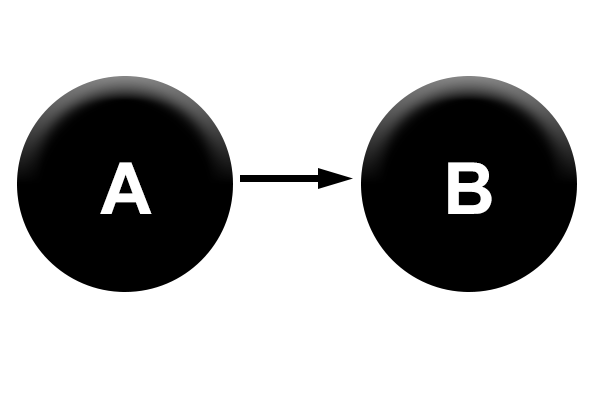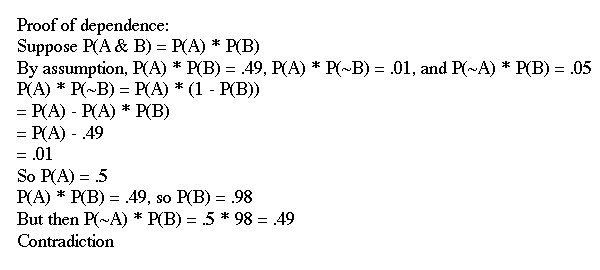# Causal Arrows

Previous post: Preliminaries

Let’s start discussing causality. The first thing I want to get across is that causal models tell us how to factor joint probability distributions.

Let’s say that we want to express a causal relationship between some variable A and another variable B. We’ll draw it this way:Let’s say that A = “It is raining”, and B = “The sidewalk is wet.”

Let’s assign probabilities to the various possibilities.

P(A & B) = 49%
P(A & ~B) = 1%
P(~A & B) = 5%
P(~A & ~B) = 45%

This is the joint probability distribution for our variables A and B. It tells us that it rains about half the time, that the sidewalk is almost always wet when it rains, and the sidewalk is rarely wet when it doesn’t rain.

Factorizations of a joint probability distribution express the joint probabilities in terms of a product of probabilities for each variable. Any given probability distribution may have multiple equivalent factorizations. So, for instance, we can factor our distribution like this:

Factorization 1:
P(A) = 50%
P(B | A) = 98%
P(B | ~A) = 10%

And we can also factor our distribution like this:

Factorization 2
P(B) = 54%
P(A | B) = 90.741%
P(A | ~B) = 2.174%

You can check for yourself that these factorizations are equivalent to our starting joint probability distribution by using the relationship between joint probabilities and conditional probabilities. For example, using Factorization 1:

P(A & ~B)
= P(A) · P(~B | A)
= 50% · 2%
= 1%

Just as expected! If any of this is confusing to you, go back to my last post.

***

Let’s rewind. What does any of this have to do with causality? Well, the diagram we drew above, in which rain causes sidewalk-wetness, instructs us as to how we should factor our joint probability distribution.

Here are the rules:

1. If node X has no incoming arrows, you express its probability as P(X).
2. If a node does have incoming arrows, you express its probability as conditional upon the values of its parent nodes – those from which the arrows originate.

Let’s look back at our diagram for rain and sidewalk-wetness.Which representation do we use?

A has no incoming arrows, so we express its probability unconditionally: P(A).

B has one incoming arrow from A, so we express its probability as conditional upon the possible values of A. That is, we use P(B | A) and P(B | ~A).

Which means that we use Factorization 1!

Say that instead somebody tells you that they think the causal relationship between rain and sidewalk-wetness goes the other way. I.e., they believe that the correct diagram is:Which factorization would they use?

***

So causal diagrams tell us how to factor a probability distribution over multiple variables. But why does this matter? After all, two different factorizations of a single probability distribution are empirically equivalent. Doesn’t this mean that “A causes B” and “B causes A” are empirically indistinguishable?

Two responses: First, this is only one component of causal models. Other uses of causal models that we will see in the next post will allow us to empirically determine the direction of causation.

And second: in fact, some causal diagrams can be empirically distinguished.

Say that somebody proclaims that there are no causal links between rain and sidewalk-wetness. We represent this as follows:What does this tell us about how to express our probability distribution?

Well, A has no incoming arrows, so we use P(A). B has no incoming arrows, so we use P(B).

So let’s say we want to know the chance that it’s raining AND the sidewalk is wet. According to the diagram, we’ll calculate this in the following way:

P(A & B) = P(A) · P(B)

But wait! Let’s look back at our initial distribution:

P(A & B) = 49%
P(A & ~B) = 1%
P(~A & B) = 5%
P(~A & ~B) = 45%

Is it possible to get all of these values from just our two values P(A) and P(B)? No! (proof below)

In other words, our data rules out this causal model.***

To summarize: a causal diagram over two variables A and B tells you how to calculate things like P(A & B). It says that you break it into the probabilities of the individual propositions, and that the probability for each variable should be conditional on the possible values of its parents.

Next we’ll look at how we can empirically distinguish betweenPrevious post: Preliminaries

Next post: Causal intervention